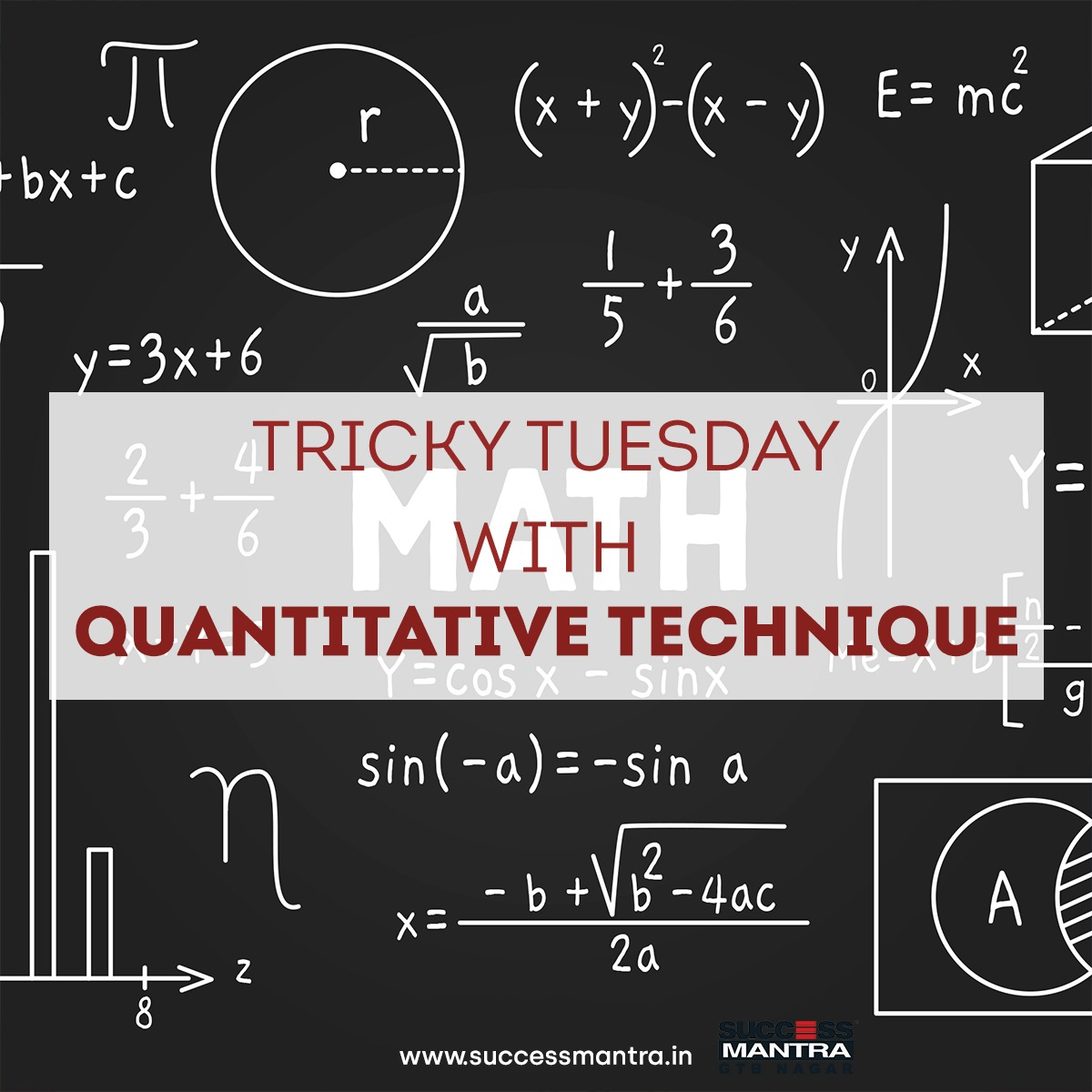## Questions On Quantitative Techniques SMQTQ0391. The product of two numbers is 120 and the sum of their squares is 289. The sum of the number is:

a) 20

b) 19

c) 23

d) None of these

2. The H.C.F. of two numbers is 23 and the other two factors of their L.C.M. are 13 and 14. The larger of the two numbers is:

a) 276

b) 322

c) 276

d) 385

3. A person takes a loan of Rs. 2500 at 4% simple interest. He returns Rs. 1500 at the end of 1 year. What amount he would pay to clear his dues at the end of 2 years?

a) Rs. 1140

b) Rs. 1150

c) Rs. 1100

d) Rs. 1050

4. A trader bought chocolates at three for a hundred rupees. How many chocolates should he sell for a hundred to gain 50%:

a) 2

b) 3

c) 4

d) 5